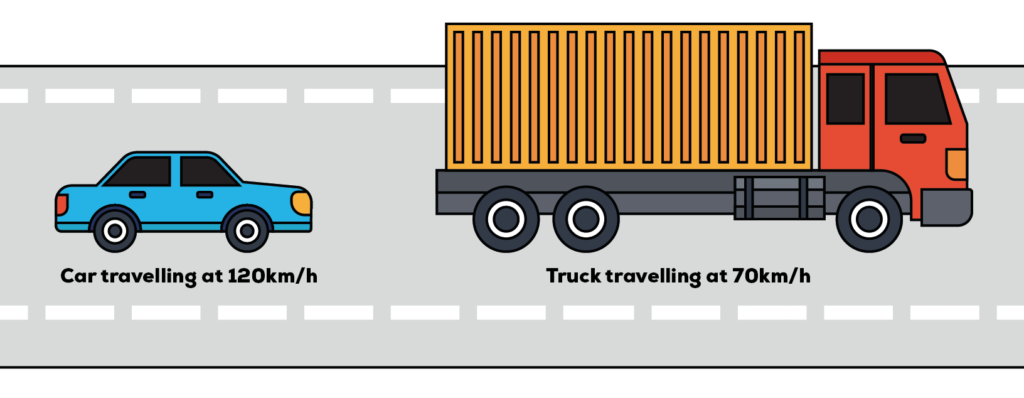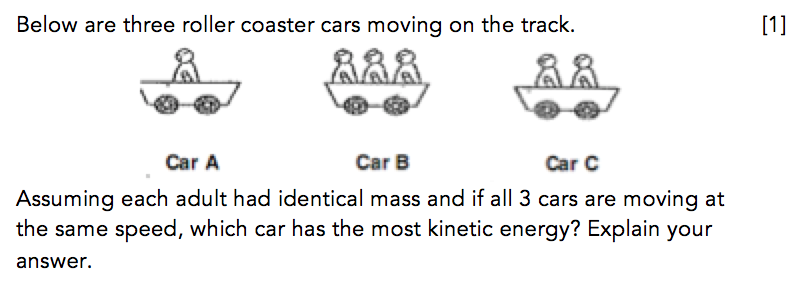In recent years, I have observed an increasing number of questions testing students on their understanding of the factors affecting Kinetic Energy.

Questions in examinations typically test students on their understanding and application of the factors affecting Gravitational Potential Energy and the Conversion of Energy. As a result, they may not be very familiar with the factors affecting Kinetic Energy.

As such, in today’s article, I will be giving you a quick introduction of Kinetic Energy and share how students can apply the factors affecting Kinetic Energy to tackle open-ended questions 🙂

## What Is Kinetic Energy & What Are The Factors Affecting It?

Kinetic Energy is energy an object possesses due to its motion. The amount of kinetic energy an object possesses depends on two factors:

### 1. Mass of the object

The greater the mass of the object, the greater the amount of kinetic energy possessed by the object.

(Example: A heavier truck travelling at 60km/h possesses more kinetic energy than a lighter car travelling at 60km/h.)

### 2. Speed Of The Object

The greater the speed of the object, the greater the amount of kinetic energy possessed by the object.

(Example: A 170kg motorcycle travelling at 100km/h will possess kinetic energy than a 170kg motorcycle travelling at 80km/h.)

## Now consider this question:

What about a 1500kg truck travelling at 70km/h and a 180kg car travelling at 120km/h?Which vehicle possesses a greater amount of kinetic energy?

We would not be able to answer this question using the primary school Science syllabus as more than one variable has changed. The car has a greater mass than the motorcycle, whereas the motorcycle has a greater speed. In order to answer questions testing on the factors affecting kinetic energy, there can only be one changed factor while the other factor must remain the same.

Next, let us now take a look at the question below:

## QuestionBVPS/2014/P6/SA1/Q37

In this question, there are a total of 3 roller coaster cars moving on the track and each car has a different number of adults sitting in it.

By keeping the speed of the cars the same and varying the number of people in the car, the question is testing students on their understanding of the factors affecting kinetic energy.

As the speed of all 3 cars is the same, the factor students should be focusing on is the different masses of the cars.

Assuming that the weight of each adult is the same, the heaviest car would be Car B and the lightest car would be Car A.

Hence, Car B would possess the most amount of kinetic energy.